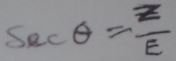Find the trigonometric ratios indicated

Otis

Elite Member
but why am i not wrong with the sec…
i did not put Sec0 =z/e
I'm not sure that I understand.

You did write sec(θ) = z/eWith the corrected labels, you have:

cos(θ) = ω/Z

sec(θ) = Z/ω

ω/Z and Z/ω are reciprocals, so you have sec(θ) = 1/cos(θ).Subhotosh Khan

Super Moderator
Staff member
Can someone please help solve this and show me how come about. This is an assignment but am yet to understand this topic: Thanks
That question is:
find the trigonometric ratios indicated

View attachment 29848

ugu

Junior Member
Not not yet but I need submit today, I will try finish and show

ugu

Junior Member
If they are reciprocals, do it mean that w/z cancels z/w ? If yes, why cant we also say cos(0) = 1/Sec(0) ? I used 0 to represent tita

Subhotosh Khan

Super Moderator
Staff member
If they are reciprocals, do it mean that w/z cancels z/w ? If yes, why cant we also say cos(0) = 1/Sec(0) ? I used 0 to represent tita
If yes, why cant we also say cos(0) = 1/Sec(0)
Yes you can - as long as $$\displaystyle sec(\theta) \ne 0$$

Otis

Elite Member
If they are reciprocals, [doesd] it mean that w/z cancels z/w ?
No. It means that you may write each as the reciprocal of the other.

If we multiply two reciprocals, we get 1. (Maybe that's what you meant by w/Z cancelling Z/w.)

You have correctly shown the identities:

sec(θ) = 1/cos(θ)

1/sec(θ) = cos(θ)ugu

Junior Member
Hi ugudansam. All answers look correct!

Two comments. Some letters 'a' look like '9'. You may want to be mindful of that. On #3, you'd written the answer for sec(β) twice. (No big deal.)

View attachment 29888

CheersSo grateful, and yes, I wrote the answer twice since the question came twice. Thanks for the tutorial as I will further the study here. However, for the formula or secant, cosecant and cotangent. I hear something like (ALL STUDENT LIKE CHEMISTRY) I wonder the application. Else it mean one just have to memorise them

Otis

Elite Member
I wrote the answer twice since the question came twice.
Indeed it does. Good on ya.

formula for secant, cosecant and cotangent ... [maybe] one just have to memorise them
Or, one could memorize cosine, sine, and tangent definitions, first. That way, you simply write their reciprocals, to get the other three. You'd only need to remember that the name secant is associated with cosine and the name cosecant is associated with sine.

When I was a beginner, I think I'd linked secant and cosine together because both names have two syllables, and that helped me remember that secant is the reciprocal of cosine.